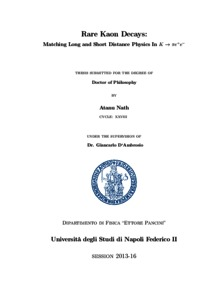Ricerca
Browse
Informazioni

Nath, Atanu (2016) Rare Kaon Decays: Matching Long And Short Distance Physics In K - > Pi e+ e-. [Tesi di dottorato]Preview
Text
Nath_Atanu_XXVIII.pdf

Download (2MB) | Preview
[error in script] [error in script]
Item Type: Tesi di dottorato
Lingua: English
Title: Rare Kaon Decays: Matching Long And Short Distance Physics In K - > Pi e+ e-
Creators:
CreatorsEmail
Nath, Atanuatanu.nath@fisica.unina.it
Date: 16 March 2016
Number of Pages: 154
Institution: Università degli Studi di Napoli Federico II
Department: Fisica
Scuola di dottorato: Scienze fisiche
Dottorato: Fisica fondamentale ed applicata
Ciclo di dottorato: 28
Coordinatore del Corso di dottorato:
nomeemail
Velotta, Raffaelevelotta@na.infn.it
Tutor:
nomeemail
D'Ambrosio, GiancarloUNSPECIFIED
Date: 16 March 2016
Number of Pages: 154
Uncontrolled Keywords: radiative kaon decays, chiral perturbation theory, large N, QCD, weak chiral lagrangian, OPE, long-short matching, hidden local symmetry, vector mesons
Settori scientifico-disciplinari del MIUR: Area 02 - Scienze fisiche > FIS/02 - Fisica teorica, modelli e metodi matematici
Area 02 - Scienze fisiche > FIS/04 - Fisica nucleare e subnucleare
Date Deposited: 14 Apr 2016 20:49
Last Modified: 31 Oct 2016 09:48
URI: http://www.fedoa.unina.it/id/eprint/10691## Abstract

In this thesis we try to understand the non-perturbative regime of QCD through long and short distance matching of the decay amplitude K->pi e^+ e^-. The first Chapter contains brief introduction and motivation then in the end we introduce the notations and transformation properties of various quantities and tables of phenomenological parameters. In the second chapter we discuss the Chiral Symmetry and its breaking and the construction of Delta S = 0,1 Lagrangians. Then we apply chiral Lagrangian to calculate the amplitude of K^+ -> pi^+ pi^- decay at one loop in section of the second chapter, the approach is a little bit different than that of Ecker, Pick and Raffael but a few tricks introduced by them were used. In section 2.7.3 we introduce the beyond leading order dispersive calculation of the same decay by D'Ambrosio et al. where the phenomenological parameters a_i and b_i (that completely fixes the form factor of the decay under study) were introduced and were predicted in the last chapter. In chapter 3 we start with a brief discussion of long and short distance matching of QCD and the calculation of Wilson coefficients. Then we introduce in reasonable detail the Bardeen, Buras and G`erard (BBG) scheme of matching which plays the central role in our work. In the last chapter we apply BBG scheme to calculate the form factor of the deacy K -> pi e^+ e^-, first in section 4.0.6 without vector meson resonances and find values which are extremely small compared to the experimental values then in section 4.1 we introduce the resonances through Hidden Local Symmetry (HLS) and construct the weak chiral Lagrangian containing vector coupling based on the Gilman-Wise Delta S = 1 Hamiltonian, we then use it to calculate the appropriate extension of the BBG long distane evolution operator introduced in chapter 3 and calculate the a_i and b_i parameters. Vector inclusion shows huge enhancements in both parameters. We provide detailed evaluations of the loop integrals in Appendix B, Feynman rules and other conventions are presented in Appendix A and in Appendix C we present the large N structure of relevant Wilson coefficients. Notations, symbols and transformation properties of quantities along with various phenomenological parameters and their values are provided in the end of the introductory chapter.